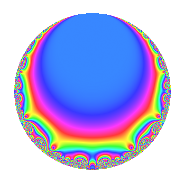# Properties

 Label 21.3.h.aLevel 21 Weight 3 Character orbit 21.h Analytic conductor 0.572 Analytic rank 0 Dimension 2 CM disc. -3 Inner twists 4

# Related objects

## Newspace parameters

 Level: $$N$$ = $$21 = 3 \cdot 7$$ Weight: $$k$$ = $$3$$ Character orbit: $$[\chi]$$ = 21.h (of order $$6$$ and degree $$2$$)

## Newform invariants

 Self dual: No Analytic conductor: $$0.572208555157$$ Analytic rank: $$0$$ Dimension: $$2$$ Coefficient field: $$\Q(\sqrt{-3})$$ Coefficient ring: $$\Z[a_1, \ldots, a_{4}]$$ Coefficient ring index: $$1$$ Sato-Tate group: $\mathrm{U}(1)[D_{6}]$

## $q$-expansion

Coefficients of the $$q$$-expansion are expressed in terms of a primitive root of unity $$\zeta_{6}$$. We also show the integral $$q$$-expansion of the trace form.

 $$f(q)$$ $$=$$ $$q$$ $$+ 3 \zeta_{6} q^{3}$$ $$-4 \zeta_{6} q^{4}$$ $$+ ( -5 - 3 \zeta_{6} ) q^{7}$$ $$+ ( -9 + 9 \zeta_{6} ) q^{9}$$ $$+O(q^{10})$$ $$q$$ $$+ 3 \zeta_{6} q^{3}$$ $$-4 \zeta_{6} q^{4}$$ $$+ ( -5 - 3 \zeta_{6} ) q^{7}$$ $$+ ( -9 + 9 \zeta_{6} ) q^{9}$$ $$+ ( 12 - 12 \zeta_{6} ) q^{12}$$ $$+ 23 q^{13}$$ $$+ ( -16 + 16 \zeta_{6} ) q^{16}$$ $$+ ( -11 + 11 \zeta_{6} ) q^{19}$$ $$+ ( 9 - 24 \zeta_{6} ) q^{21}$$ $$-25 \zeta_{6} q^{25}$$ $$-27 q^{27}$$ $$+ ( -12 + 32 \zeta_{6} ) q^{28}$$ $$+ 13 \zeta_{6} q^{31}$$ $$+ 36 q^{36}$$ $$+ ( 73 - 73 \zeta_{6} ) q^{37}$$ $$+ 69 \zeta_{6} q^{39}$$ $$-61 q^{43}$$ $$-48 q^{48}$$ $$+ ( 16 + 39 \zeta_{6} ) q^{49}$$ $$-92 \zeta_{6} q^{52}$$ $$-33 q^{57}$$ $$+ ( -74 + 74 \zeta_{6} ) q^{61}$$ $$+ ( 72 - 45 \zeta_{6} ) q^{63}$$ $$+ 64 q^{64}$$ $$+ 13 \zeta_{6} q^{67}$$ $$+ 97 \zeta_{6} q^{73}$$ $$+ ( 75 - 75 \zeta_{6} ) q^{75}$$ $$+ 44 q^{76}$$ $$+ ( -11 + 11 \zeta_{6} ) q^{79}$$ $$-81 \zeta_{6} q^{81}$$ $$+ ( -96 + 60 \zeta_{6} ) q^{84}$$ $$+ ( -115 - 69 \zeta_{6} ) q^{91}$$ $$+ ( -39 + 39 \zeta_{6} ) q^{93}$$ $$+ 2 q^{97}$$ $$+O(q^{100})$$ $$\operatorname{Tr}(f)(q)$$ $$=$$ $$2q$$ $$\mathstrut +\mathstrut 3q^{3}$$ $$\mathstrut -\mathstrut 4q^{4}$$ $$\mathstrut -\mathstrut 13q^{7}$$ $$\mathstrut -\mathstrut 9q^{9}$$ $$\mathstrut +\mathstrut O(q^{10})$$ $$2q$$ $$\mathstrut +\mathstrut 3q^{3}$$ $$\mathstrut -\mathstrut 4q^{4}$$ $$\mathstrut -\mathstrut 13q^{7}$$ $$\mathstrut -\mathstrut 9q^{9}$$ $$\mathstrut +\mathstrut 12q^{12}$$ $$\mathstrut +\mathstrut 46q^{13}$$ $$\mathstrut -\mathstrut 16q^{16}$$ $$\mathstrut -\mathstrut 11q^{19}$$ $$\mathstrut -\mathstrut 6q^{21}$$ $$\mathstrut -\mathstrut 25q^{25}$$ $$\mathstrut -\mathstrut 54q^{27}$$ $$\mathstrut +\mathstrut 8q^{28}$$ $$\mathstrut +\mathstrut 13q^{31}$$ $$\mathstrut +\mathstrut 72q^{36}$$ $$\mathstrut +\mathstrut 73q^{37}$$ $$\mathstrut +\mathstrut 69q^{39}$$ $$\mathstrut -\mathstrut 122q^{43}$$ $$\mathstrut -\mathstrut 96q^{48}$$ $$\mathstrut +\mathstrut 71q^{49}$$ $$\mathstrut -\mathstrut 92q^{52}$$ $$\mathstrut -\mathstrut 66q^{57}$$ $$\mathstrut -\mathstrut 74q^{61}$$ $$\mathstrut +\mathstrut 99q^{63}$$ $$\mathstrut +\mathstrut 128q^{64}$$ $$\mathstrut +\mathstrut 13q^{67}$$ $$\mathstrut +\mathstrut 97q^{73}$$ $$\mathstrut +\mathstrut 75q^{75}$$ $$\mathstrut +\mathstrut 88q^{76}$$ $$\mathstrut -\mathstrut 11q^{79}$$ $$\mathstrut -\mathstrut 81q^{81}$$ $$\mathstrut -\mathstrut 132q^{84}$$ $$\mathstrut -\mathstrut 299q^{91}$$ $$\mathstrut -\mathstrut 39q^{93}$$ $$\mathstrut +\mathstrut 4q^{97}$$ $$\mathstrut +\mathstrut O(q^{100})$$

## Character Values

We give the values of $$\chi$$ on generators for $$\left(\mathbb{Z}/21\mathbb{Z}\right)^\times$$.

 $$n$$ $$8$$ $$10$$ $$\chi(n)$$ $$-1$$ $$-1 + \zeta_{6}$$

## Embeddings

For each embedding $$\iota_m$$ of the coefficient field, the values $$\iota_m(a_n)$$ are shown below.

For more information on an embedded modular form you can click on its label.

Label $$\iota_m(\nu)$$ $$a_{2}$$ $$a_{3}$$ $$a_{4}$$ $$a_{5}$$ $$a_{6}$$ $$a_{7}$$ $$a_{8}$$ $$a_{9}$$ $$a_{10}$$
2.1
 0.5 + 0.866025i 0.5 − 0.866025i
0 1.50000 + 2.59808i −2.00000 3.46410i 0 0 −6.50000 2.59808i 0 −4.50000 + 7.79423i 0
11.1 0 1.50000 2.59808i −2.00000 + 3.46410i 0 0 −6.50000 + 2.59808i 0 −4.50000 7.79423i 0
 $$n$$: e.g. 2-40 or 990-1000 Significant digits: Format: Complex embeddings Normalized embeddings Satake parameters Satake angles

## Inner twists

Char. orbit Parity Mult. Self Twist Proved
1.a Even 1 trivial yes
3.b Odd 1 CM by $$\Q(\sqrt{-3})$$ yes
7.c Even 1 yes
21.h Odd 1 yes

## Hecke kernels

This newform can be constructed as the kernel of the linear operator $$T_{2}$$ acting on $$S_{3}^{\mathrm{new}}(21, [\chi])$$.